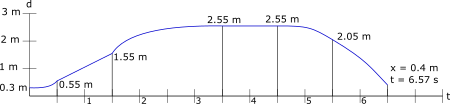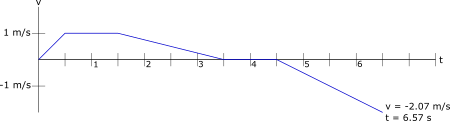# For the following described motion, draw a position-time, a velocitytime, and an...

## Question:

For the following described motion, draw a position-time, a velocitytime, and an acceleration-time graph on the grids provided. You may find it easier to do most of the velocity-time graph first, since you are given all but the last time, then do whatever calculations you need to to make sure the other two graphs are consistent with it, and get the final time. Show all your work for calculating exact positions, velocities and times. The graphs should be exact. Take ?away from the detector? as the positive direction.

1. You begin at rest 0.3m in front of the detector and walk away from the detector steadily speeding up for 0.5 seconds. In this time, you go from rest to 1.0m/s.

2. You walk away from the detector steadily at 1.0m/s for 1.0 second.

3. You come to rest steadily over a 2.0 second period. 1

4. You stand still for 1.0 second.

5. You walk toward the detector speeding up steadily for 1.0 second, going from rest to 1.0m/s.

6. You walk toward the detector continuing at this steady rate until you return to the 0.4 meter position.

(a) Draw the position-time graph of the motion.

(b) Draw the velocity-time graph of the motion.

(c) Draw the acceleration-time graph for the motion.

## Kinematics: Displacement, Velocity and Acceleration Graphs

In this problem, we will use the following kinematics equations to solve for the time, velocity, position, and acceleration given different scenarios.

The equations that we will use are listed below:

{eq}v = v_{0} + at\\ \Delta x = \left ( \dfrac{v + v_{0}}{2} \right )t\\ \Delta x = v_{0}t + \frac{1}{2}at^{2}\\ v^{2} = v_{0}^{2} + 2a\Delta x{/eq}

After we obtain the needed values for each step, we will then carefully graph the displacement vs. time, velocity vs. time and acceleration vs. time for the whole motion.1. You begin at rest 0.3m in front of the detector and walk away from the detector steadily speeding up for 0.5 seconds. In this time, you go from rest to 1.0m/s.

Time (s) Velocity (m/s) Position (m)
0 0 0.3
0.5 +1.00 Xf

{eq}a = \left ( \dfrac{v - v_{0}}{t} \right )\\ a = \left ( \dfrac{1 \frac{m}{s} - 0 \frac{m}{s}}{0.5 s - 0s} \right )\\ a = 2 \dfrac{m}{s^{2}}{/eq}

{eq}x_{f} = x_{0} + v_{0}t + \frac{1}{2}at^{2}\\ x_{f} = 0.3m + 0 + \frac{1}{2}\left (2 \frac{m}{s^{2}} \right )(0.5s)^{2}\\ x_{f} = 0.55 m{/eq}

2. You walk away from the detector steadily at 1.0m/s for 1.0 second.

Time (s) Velocity (m/s) Position (m)
0.5 +1.00 0.55
1.5 +1.00 Xf

{eq}a = \left ( \dfrac{v - v_{0}}{t} \right )\\ a = \left ( \dfrac{1 \frac{m}{s} - 1 \frac{m}{s}}{1.5 s - 0.5s} \right )\\ a = 0 \dfrac{m}{s^{2}}{/eq}

{eq}x_{f} = x_{0} + v_{0}t + \frac{1}{2}at^{2}\\ x_{f} = 0.55m + (1\frac{m}{s})(1s) + \frac{1}{2}\left (0 \frac{m}{s^{2}} \right )(1s)^{2}\\ x_{f} = 1.55 m{/eq}

3. You come to rest steadily over a 2.0 second period. 1

Time (s) Velocity (m/s) Position (m)
1.5 +1.00 1.55
3.5 0 Xf

{eq}a = \left ( \dfrac{v - v_{0}}{t} \right )\\ a = \left ( \dfrac{0 \frac{m}{s} - 1 \frac{m}{s}}{3.5 s - 1.5s} \right )\\ a = -0.5 \dfrac{m}{s^{2}}{/eq}

{eq}x_{f} = x_{0} + v_{0}t + \frac{1}{2}at^{2}\\ x_{f} = 1.55m + (1\frac{m}{s})(2s) + \frac{1}{2}\left (-0.5 \frac{m}{s^{2}} \right )(2s)^{2}\\ x_{f} = 2.55 m{/eq}

4. You stand still for 1.0 second.

Time (s) Velocity (m/s) Position (m)
3.5 0 2.55
4.5 0 Xf

{eq}a = \left ( \dfrac{v - v_{0}}{t} \right )\\ a = \left ( \dfrac{0 \frac{m}{s} - 0 \frac{m}{s}}{4.5 s - 3.5s} \right )\\ a = 0 \dfrac{m}{s^{2}}{/eq}

{eq}x_{f} = x_{0} + v_{0}t + \frac{1}{2}at^{2}\\ x_{f} = 2.55m + (0\frac{m}{s})(1s) + \frac{1}{2}\left (0\frac{m}{s^{2}} \right )(1s)^{2}\\ x_{f} = 2.55 m{/eq}

5. You walk toward the detector speeding up steadily for 1.0 second, going from rest to 1.0m/s.

Time (s) Velocity (m/s) Position (m)
4.5 0 2.55
5.5 -1.00 Xf

{eq}a = \left ( \dfrac{v - v_{0}}{t} \right )\\ a = \left ( \dfrac{-1.0 \frac{m}{s} - 0 \frac{m}{s}}{5.5 s - 4.5s} \right )\\ a = -1.00 \dfrac{m}{s^{2}}{/eq}

{eq}x_{f} = x_{0} + v_{0}t + \frac{1}{2}at^{2}\\ x_{f} = 2.55m + (-1.00\frac{m}{s})(1s) + \frac{1}{2}\left (-1.00\frac{m}{s^{2}} \right )(1s)^{2}\\ x_{f} = 2.05 m{/eq}

6. You walk toward the detector continuing at this steady rate until you return to the 0.4 meter position.

Time (s) Velocity (m/s) Position (m) Acceleration (m/s^2)
5.5 -1.00 2.05 -1.00
tf vf 0.4

{eq}x_{f} = x_{0} + v_{0}t + \frac{1}{2}at^{2}\\ 4.0m = 2.05m + (-1.00\frac{m}{s})(t) + \frac{1}{2}\left (-1.00\frac{m}{s^{2}} \right )(t)^{2}\\ 0 = -\frac{1}{2}t^{2} - t +1.65\\ t = 1.0736 s{/eq}

{eq}v_{f} = v_{0} + at\\ v_{f} = (-1\frac{m}{s})(1.0736s) + \left (-1.00 \frac{m}{s^{2}} \right )\\ v_{f} = -2.0736 \frac{m}{s}{/eq}

#### (a) Draw the position-time graph of the motion.#### (b) Draw the velocity-time graph of the motion.#### (c) Draw the acceleration-time graph for the motion.